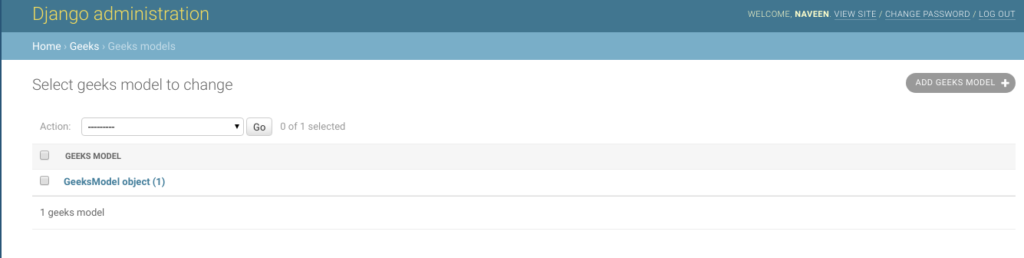# CharField – Django Models

• Last Updated : 12 Apr, 2022

CharField is a string field, for small- to large-sized strings. It is like a string field in C/C+++. CharField is generally used for storing small strings like first name, last name, etc. To store larger text TextField is used. The default form widget for this field is TextInput.

CharField has one extra required argument:

`CharField.max_length`

The maximum length (in characters) of the field. The max_length is enforced at the database level and in Django’s validation using MaxLengthValidator.

Syntax:

`field_name = models.CharField(max_length=200, **options) `

## Django Model CharField Explanation

Illustration of CharField using an Example. Consider a project named geeksforgeeks having an app named geeks.

Refer to the following articles to check how to create a project and an app in Django.

Enter the following code into models.py file of geeks app.

## Python3

 `from` `django.db ``import` `models``from` `django.db.models ``import` `Model``# Create your models here.` `class` `GeeksModel(Model):``    ``geeks_field ``=` `models.CharField(max_length ``=` `200``)`

Add the geeks app to INSTALLED_APPS

## Python3

 `# Application definition` `INSTALLED_APPS ``=` `[``    ``'django.contrib.admin'``,``    ``'django.contrib.auth'``,``    ``'django.contrib.contenttypes'``,``    ``'django.contrib.sessions'``,``    ``'django.contrib.messages'``,``    ``'django.contrib.staticfiles'``,``    ``'geeks'``,``]`

Now when we run makemigrations command from the terminal,

`Python manage.py makemigrations`

A new folder named migrations would be created in geeks directory with a file named 0001_initial.py

## Python3

 `# Generated by Django 2.2.5 on 2019-09-25 06:00` `from` `django.db ``import` `migrations, models` `class` `Migration(migrations.Migration):` `    ``initial ``=` `True` `    ``dependencies ``=` `[``    ``]` `    ``operations ``=` `[``        ``migrations.CreateModel(``            ``name ``=``'GeeksModel'``,``            ``fields ``=``[``                ``(``'id'``,``                  ``models.AutoField(``                  ``auto_created ``=` `True``,``                  ``primary_key ``=` `True``,``                  ``serialize ``=` `False``,``                  ``verbose_name ``=``'ID'``                ``)),``                ``(``'geeks_field'``,``                  ``models.CharField(``                  ``max_length ``=` `200``,``                ``)),``            ``],``        ``),``    ``]`

Now run,

`Python manage.py migrate`

Thus, an geeks_field CharField is created when you run migrations on the project. It is a field to store small- to large-sized strings.

## How to use CharField ?

CharField is used for storing small sized strings in the database. One can store First Name, Last Name, Address Details, etc. CharField should be given an argument max_length for specifying the maximum length of string it is required to store. In production server, after the Django application is deployed, space is very limited. So it is always optimal to use max_length according to the requirement of the field. Let us create an instance of the CharField we created and check if it is working.

## Python3

 `# importing the model``# from geeks app``from` `geeks.models ``import` `GeeksModel` `# creating a instance of``# GeeksModel``geek_object ``=` `GeeksModel.objects.create(geeks_field ``=``"GFG is Best"``)``geek_object.save()`

Now let’s check it in admin server. We have created an instance of GeeksModel.## Field Options

Field Options are the arguments given to each field for applying some constraint or imparting a particular characteristic to a particular Field. For example, adding an argument null = True to CharField will enable it to store empty values for that table in a relational database.
Here are the field options and attributes that an CharField can use.

My Personal Notes arrow_drop_up